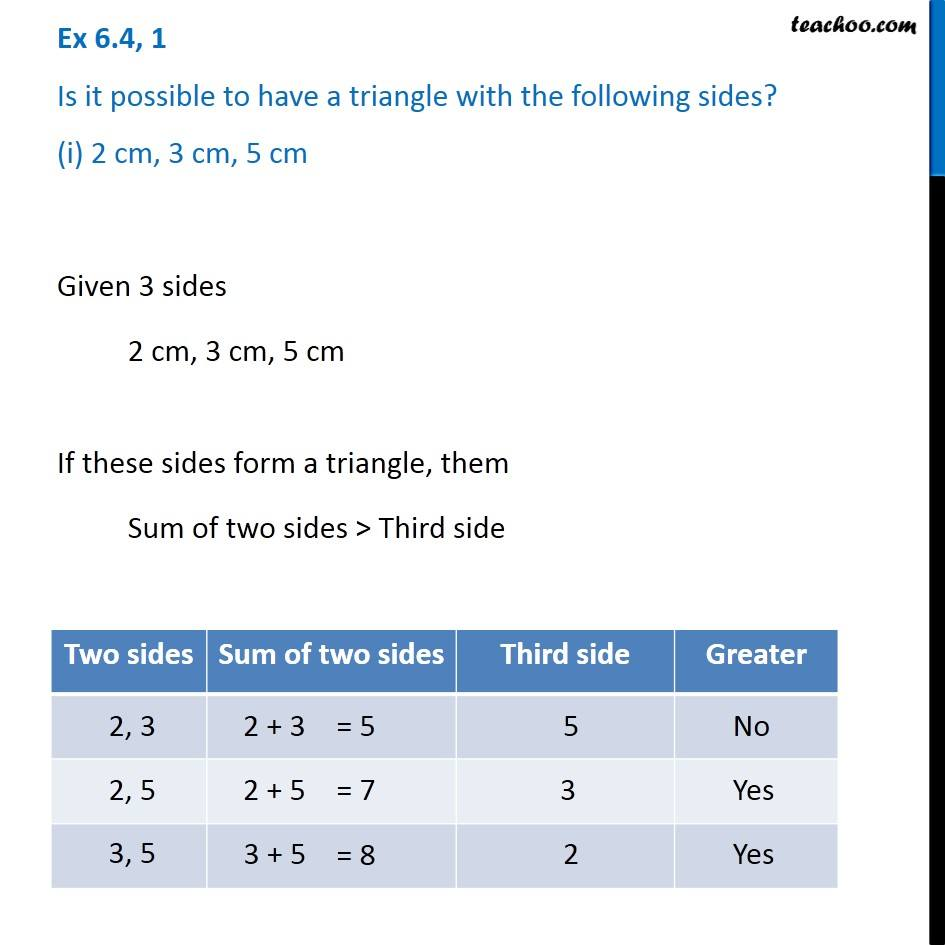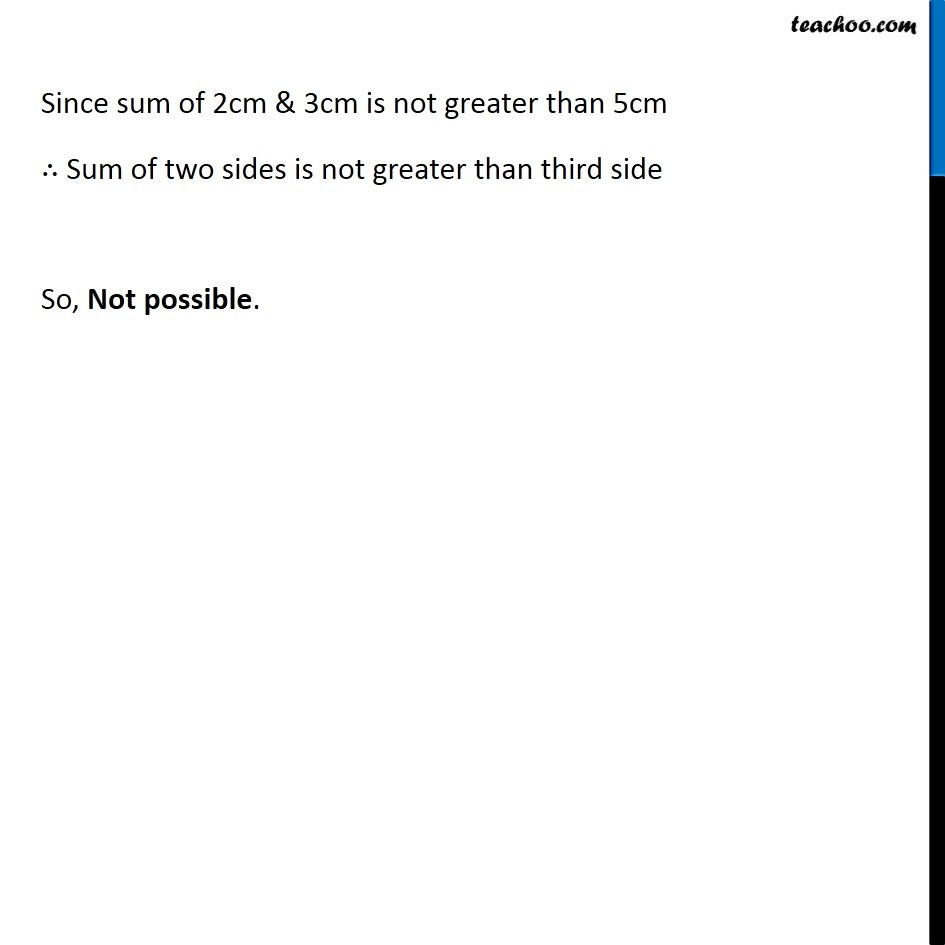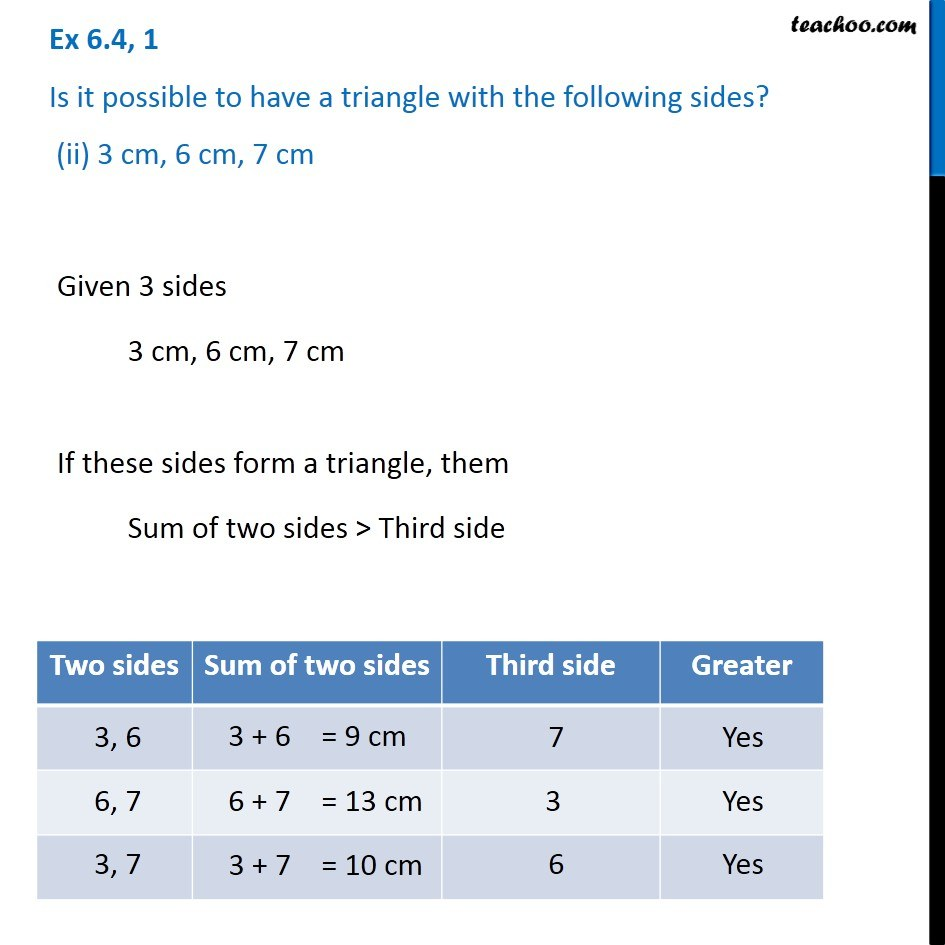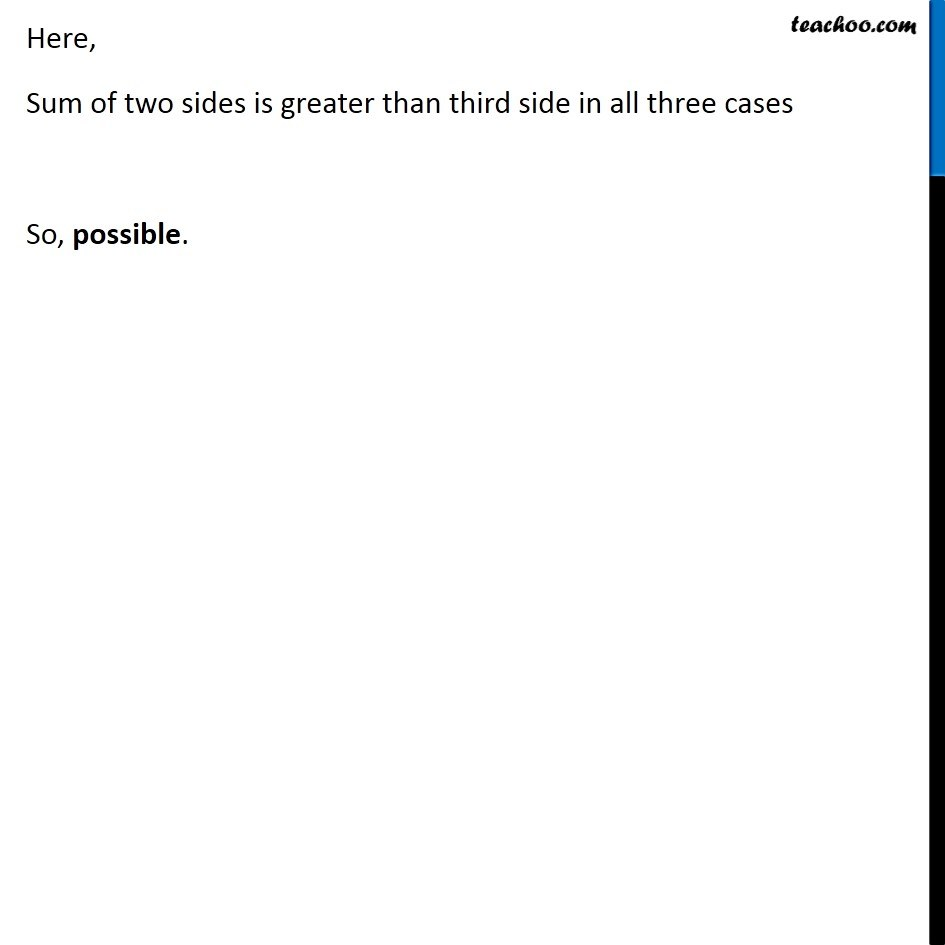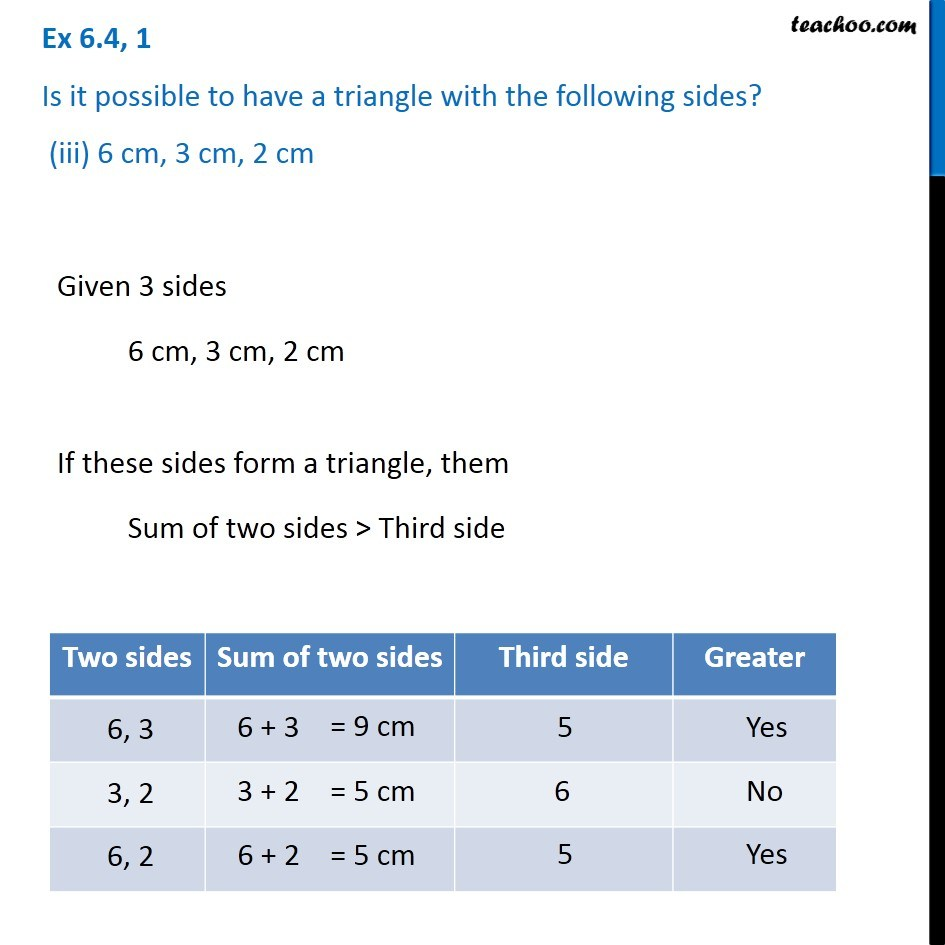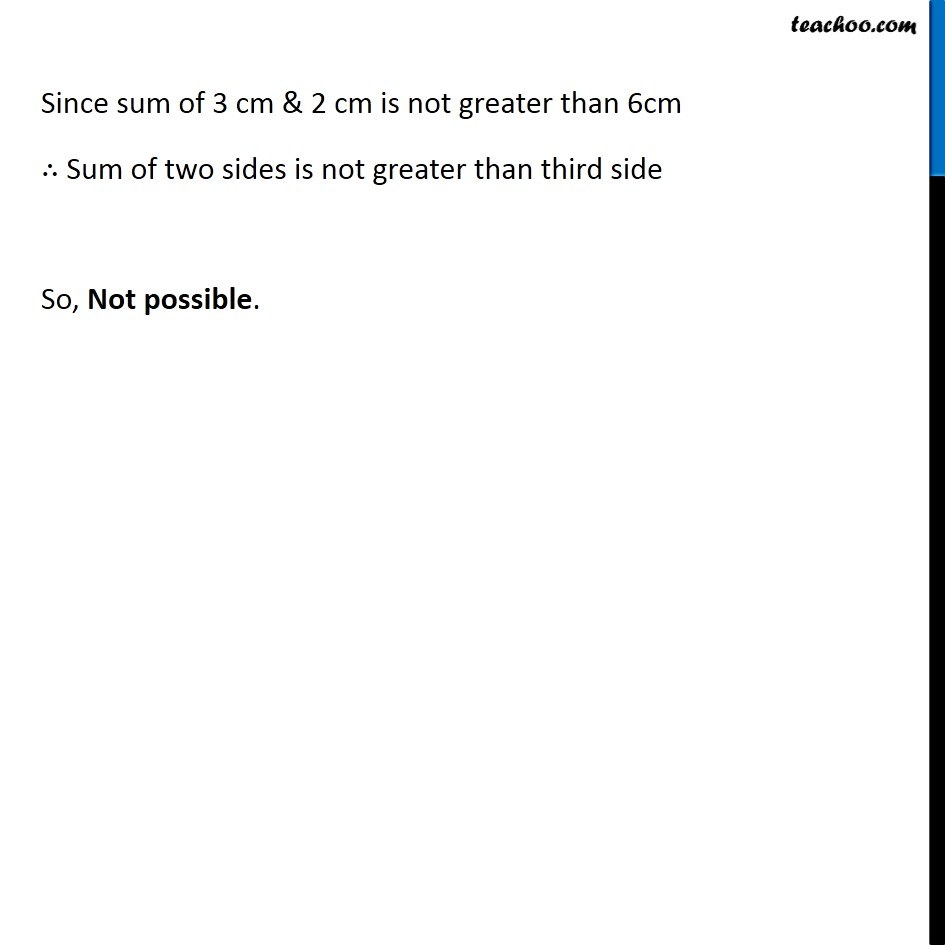1. Chapter 6 Class 7 Triangle and its Properties
2. Concept wise
3. Sum of lengths of two sides of a triangle

Transcript

Ex 6.4, 1 Is it possible to have a triangle with the following sides? (i) 2 cm, 3 cm, 5 cm Given 3 sides 2 cm, 3 cm, 5 cm If these sides form a triangle, them Sum of two sides > Third side Since sum of 2cm & 3cm is not greater than 5cm ∴ Sum of two sides is not greater than third side So, Not possible. Ex 6.4, 1 Is it possible to have a triangle with the following sides? (ii) 3 cm, 6 cm, 7 cmGiven 3 sides 3 cm, 6 cm, 7 cm If these sides form a triangle, them Sum of two sides > Third side Here, Sum of two sides is greater than third side in all three cases So, possible. Ex 6.4, 1 Is it possible to have a triangle with the following sides? (iii) 6 cm, 3 cm, 2 cm Given 3 sides 6 cm, 3 cm, 2 cm If these sides form a triangle, them Sum of two sides > Third side Since sum of 3 cm & 2 cm is not greater than 6cm ∴ Sum of two sides is not greater than third side So, Not possible.

Sum of lengths of two sides of a triangle